# Beginner's Cookbook

Author(s): TC25
In this tutorial, we will introduce several types of geospatial data, and enumerate key Earth Engine functions for analyzing and visualizing them. This cookbook was originally created as a workshop during Yale-NUS Data 2.0 hackathon, and later updated for Yale GIS Day 2018 and 2019.

## Introduction

GIS or Geographic Information System is the collection, visualization, and analysis of geographical or spatial data. In this section, we will cover the data types commonly used in GIS applications.

## Vector data

Vector data represent objects on the Earth's surface using their longitude and latitude, as well as combinations of the pairs of coordinates (lines, polylines, polygons, etc.).

### Point data

A pair of coordinates (longitude, latitude), that represents the location of points on the Earth's surface.

Example: Location of drop boxes, landmarks, etc.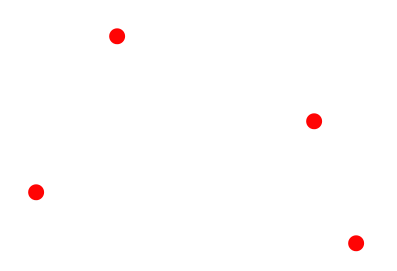### Lines

A series of points that represents a line (straight or otherwise) on the Earth's surface.

Example: Center of roads, rivers, etc.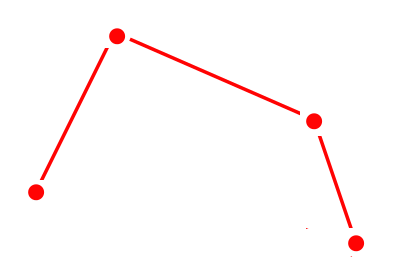### Polygons

A series of points (vertices) that define the outer edge of a region. Example: Outlines of cities, countries, continents, etc.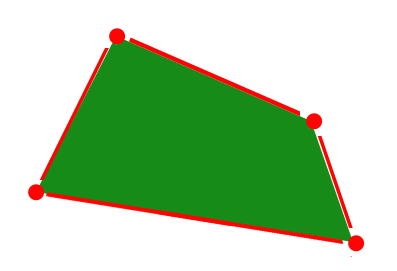## Raster data

Raster data represent objects/variables on the Earth's surface as a matrix of values, in the form of pixels, cells, or grids.

### Layers and bands

A raster is an image with a matrix of values representing the values of some observed attribute. Bands of a raster correspond to different variables, usually using the same matrix structure.

Example: Spatial variability of temperature, elevation, rainfall, etc. over a region.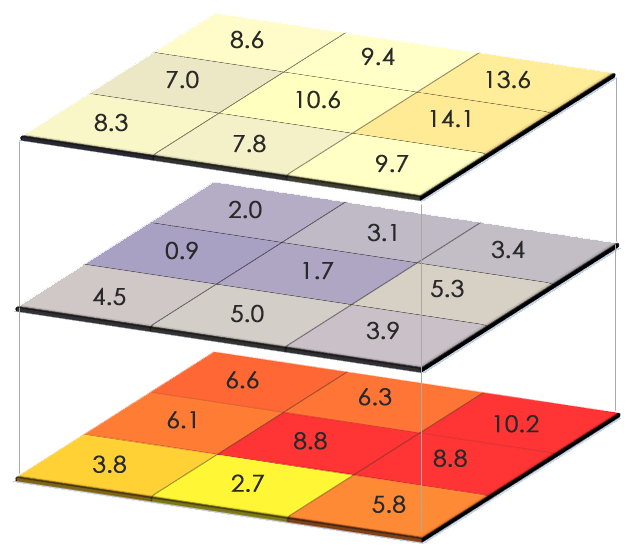Conceptual figures sourced from GISGeography

## The Google Earth Engine platform

Introductory video

Code editor

What is Earth Engine?

• A cloud-based platform for planetary scale geospatial analysis
• Uses Google's computational resources to reduce processing time
• A massive archive of remote sensing data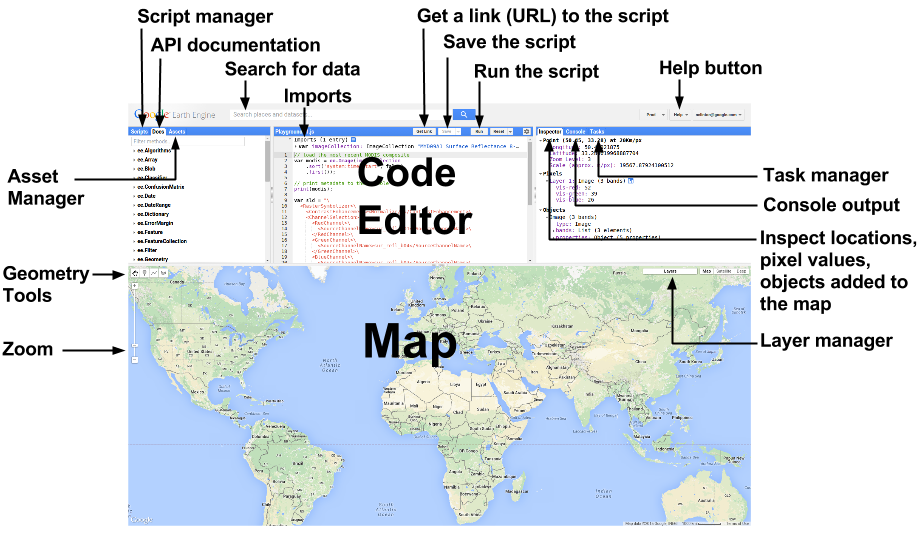## Basic functions

### Declaring variables

``````var variableName = ee.ContainerType(value);
``````

A container object (usually in the form `ee.SomeVariableType`) is used to wrap a native JavaScript object so that Google's servers can perform operations on it.

### Centering the map

``````Map.setCenter(long, lat, zoomLevel);
``````

Zoom level varies from 0 (no zoom) to 20 (highest zoom level)

``````print(variableName);
``````

The `print` operation is also useful for printing data and getting debugging info. Note: You cannot print more than 5,000 elements at once.

### Adding a layer to the map

``````Map.addLayer(variableName);
``````

## Common Earth Engine data types

### Strings

``````var str = ee.String('This is a string. Or is it? It is.');
``````

### Numbers

``````var num = ee.Number(5);
``````

### Arrays

``````var arr = ee.Array([[5, 2, 3], [-2, 7, 10], [6, 6, 9]]);
``````

### Lists

``````var lis = ee.List([5, 'five', 6, 'six']);
``````

### Dictionaries

``````var dict = ee.Dictionary({five: 5, six: 6});
``````

### And the fun stuff...

• `ee.Geometry`
• `ee.Feature`
• `ee.FeatureCollection`
• `ee.Image`
• `ee.ImageCollection`

## Declaring geometries

### Points

``````var poi = ee.Geometry.Point(0, 45);
``````

### Multi points

``````var multi = ee.Geometry.MultiPoint(0, 45, 5, 6, 70, -56);
``````

### Line string

``````var lineStr = ee.Geometry.LineString([[0, 45], [5, 6], [70, -56]]);
``````

### Multi-line string

``````var mLineStr =
ee.Geometry.MultiLineString(
[[[0, 45], [5, 6], [70, -56]], [[0, -45], [-5, -6], [-70, 56]]]);
``````

### Linear ring

``````var linRin = ee.Geometry.LinearRing(0, 45, 5, 6, 70, -56, 0, 45);
``````

### Rectangle

``````var rect = ee.Geometry.Rectangle(0, 0, 60, 30);
``````

### Polygon

``````var poly = ee.Geometry.Polygon([[[0, 0], [6, 3], [5, 5], [-30, 2], [0, 0]]]);
``````

### Multi-polygon

``````var multiPoly =
ee.Geometry.MultiPolygon(
[ee.Geometry.Polygon([[0, 0], [6, 3], [5, 5], [-30, 2], [0, 0]]),
ee.Geometry.Polygon([[0, 0], [-6, -3], [-5, -5], [30, -2], [0, 0]])]);
``````

## Features and FeatureCollections

• Features are geometries associated with specific properties.
• Feature collections are groups of features.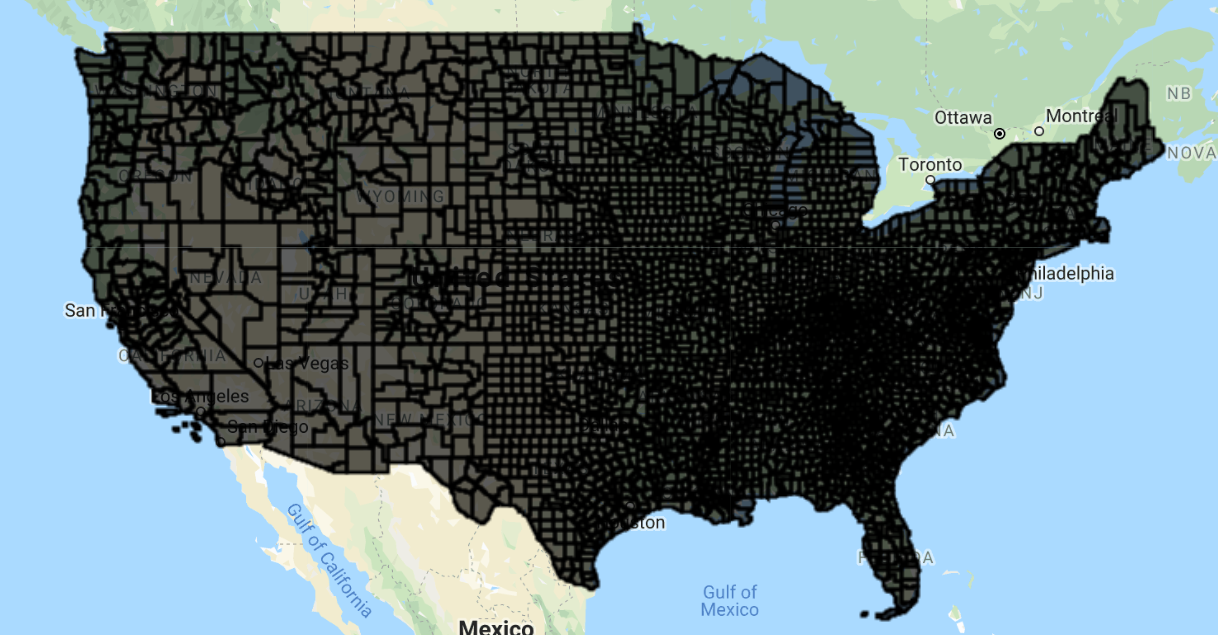## Functions and mapping

A function is a set of instructions to perform a specific task:

``````function functionName(Arguments) {
statements;
};
``````

### Calling a function

``````var result = functionName(input);
``````

### Mapping a function over a collection

``````var result = input.map(functionName);
``````

Mapping a function over a collection applies the function to every element in the collection.

## Common operations on geometries

### Finding the area of a geometry

``````var geoArea = geometry.area(maxError);
``````

By default, all units in Earth Engine are in meters.

### Finding the length of a line

``````var linLen = lineString.length(maxError);
``````

### Finding the perimeter of a geometry

``````var geoPeri = geometry.perimeter(maxError);
``````

### Reducing number of vertices in geometry

``````var simpGeo = geometry.simplify(maxError);
``````

### Finding the centroid of a geometry

``````var centrGeo = geometry.centroid(maxError);
``````

### Creating buffer around a geometry

``````var buffGeo = geometry.buffer(radius, maxError);
``````

### Finding the bounding rectangle of a geometry

``````var bounGeo = geometry.bounds(maxError);
``````

### Finding the smallest polygon that can envelope a geometry

``````var convexGeo = geometry.convexHull(maxError);
``````

### Finding common areas between two or more geometries

``````var interGeo = geometry1.intersection(geometry2, maxError);
``````

### Finding the area that includes two or more geometries

``````var unGeo = geometry1.union(geometry2, maxError);
``````

#### Example: Geometry operations

Let's run some these operations over the state of Connecticut, US using geometries of the public US counties feature collection available on Earth Engine:

1. We begin by zooming to the region of interest and loading/creating the geometries of interest by extracting them from the corresponding features.

``````// Set map center over the state of CT.
Map.setCenter(-72.6978, 41.6798, 8);
var countyData = ee.FeatureCollection('TIGER/2018/Counties');
// Filter the counties that are in Connecticut (more on filters later).
var countyConnect = countyData.filter(ee.Filter.eq('STATEFP', '09'));
// Get the union of all the county geometries in Connecticut.
var countyConnectDiss = countyConnect.union(100);
// Create a circular area using the first county in the Connecticut
// FeatureCollection.
var circle = ee.Feature(countyConnect.first())
.geometry().centroid(100).buffer(50000, 100);
// Add the layers to the map with a specified color and layer name.
``````

2. Using the `bounds()` function, we can find the rectangle that emcompasses the southernmost, westernmost, easternmost, and northernmost points of the geometry.

``````var bound = countyConnectDiss.geometry().bounds(100);
// Add the layer to the map with a specified color and layer name.
``````

3. In the same vein, but not restricting ourselves to a rectangle, a convex hull (`convexHull()`) is a polygon covering the extremities of the geometry.

``````var convex = countyConnectDiss.geometry().convexHull(100);
// Add the layer to the map with a specified color and layer name.
``````

4. Moving on to some basic operations to combine multiple geometries, the intersection (`intersection()`) is the area common to two or more geometries.

``````var intersect = convex.intersection(circle, 100);
// Add the layer to the map with a specified color and layer name.
Map.addLayer(intersect, {color: 'green'}, 'Circle and convex intersection');
``````

5. The union (`union()`) is the area encompassing two or more features.

``````// number is the maximum error in meters.
var union = convex.union(circle, 100);
// Add the layer to the map with a specified color and layer name.
Map.addLayer(union, {color: 'purple'}, 'Circle and convex union');
``````

6. We can also find the spatial difference (`difference()`) between two geometries.

``````var diff = convex.difference(circle, 100);
// Add the layer to the map with a specified color and layer name.
Map.addLayer(diff, {color: 'brown'}, 'Circle and convex difference');
``````
Difference Union Intersection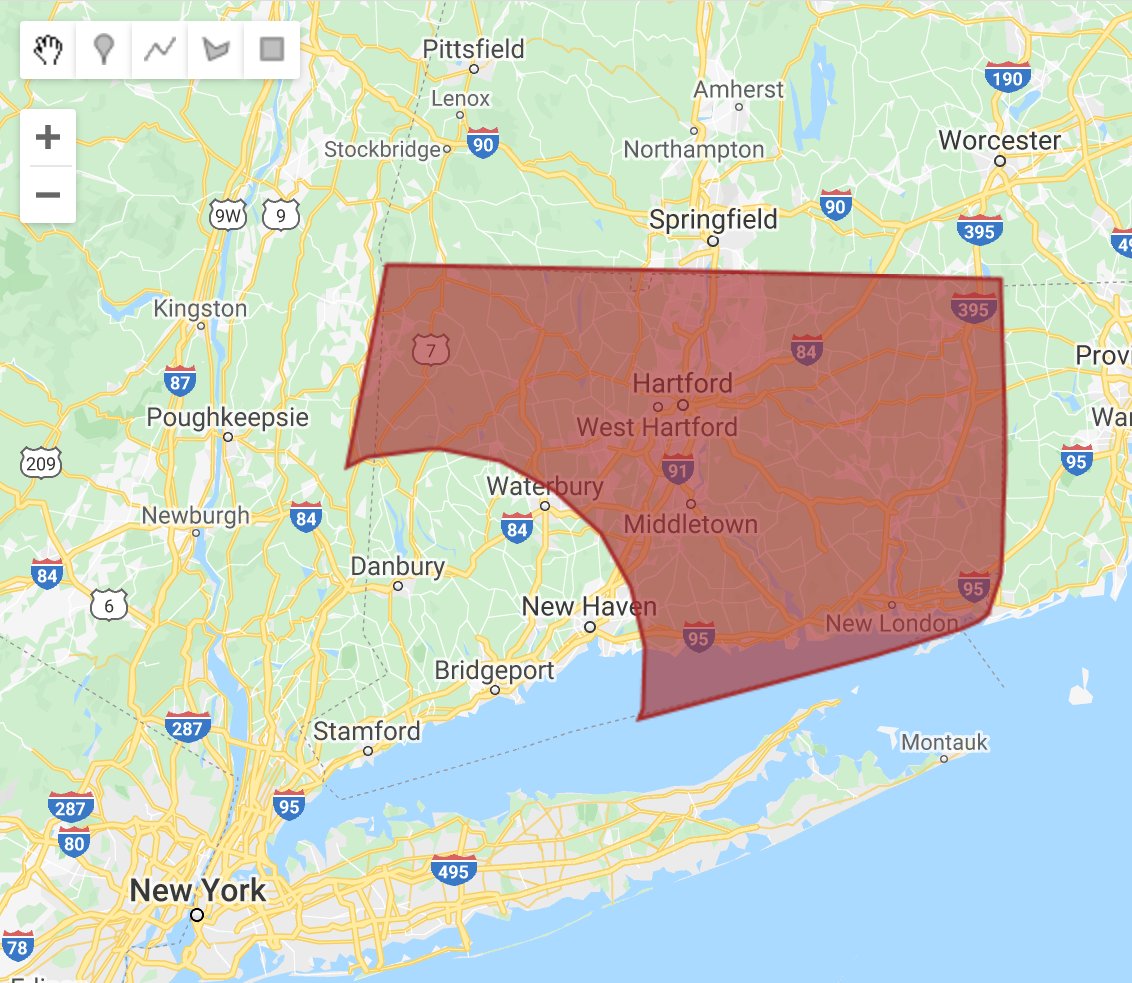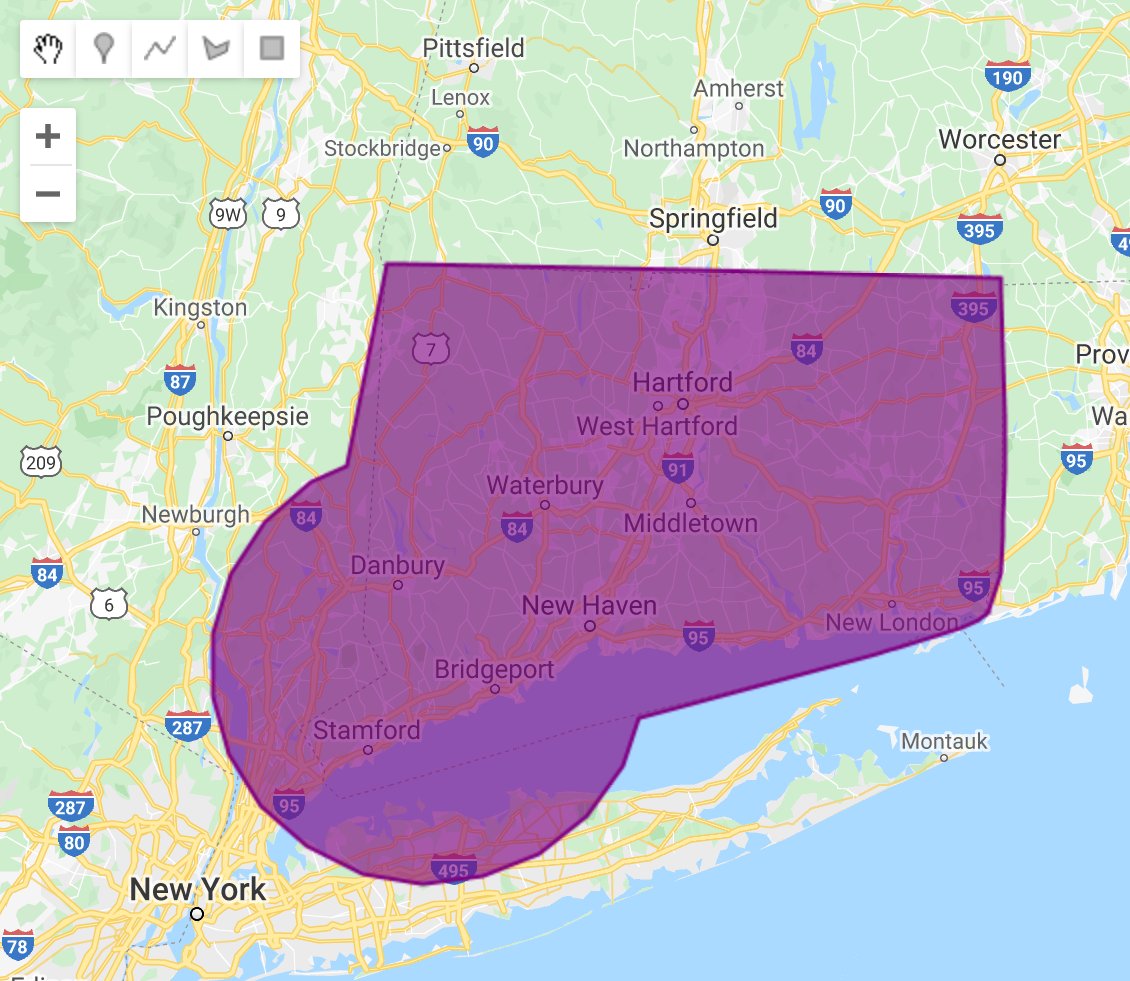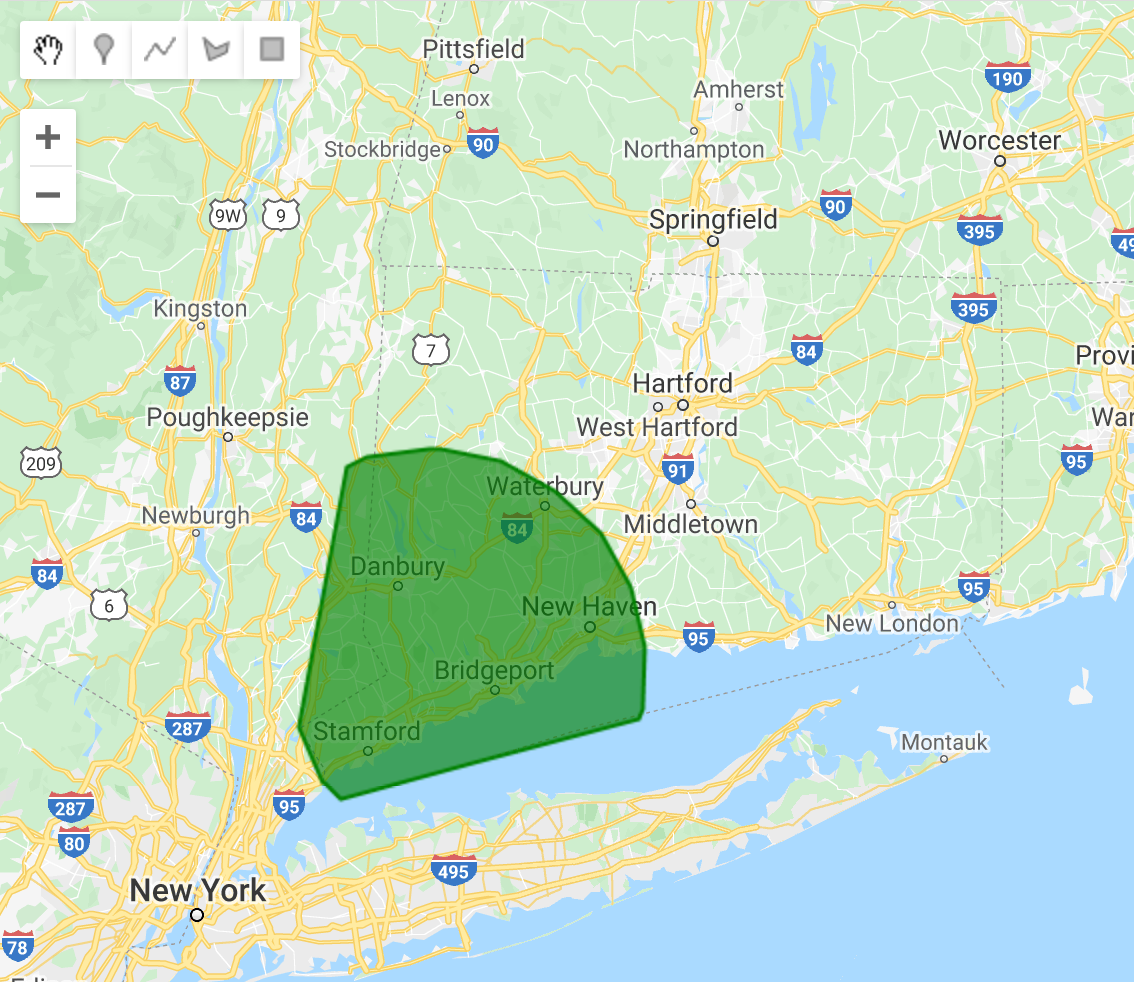7. Finally, we can calculate and display the area, length, perimeter, etc. of our geometries.

``````// Find area of feature.
var ar = countyConnectDiss.geometry().area(100);
print(ar);
// Find length of line geometry (You get zero since this is a polygon).
var length = countyConnectDiss.geometry().length(100);
print(length);
// Find perimeter of feature.
var peri = countyConnectDiss.geometry().perimeter(100);
print(peri);
``````

#### Example: Mapping over a feature collection

By mapping over a collection, one can apply the same operation on every element in a collection. For instance, let's run the same geometry operations on every county in Connecticut:

1. Similar to the previous example, we start by zooming into the map and loading the feature collection of CT counties.

``````// Set map center over the state of CT.
Map.setCenter(-72.6978, 41.6798, 8);
var countyData = ee.FeatureCollection('TIGER/2018/Counties');
// Filter the counties that are in Connecticut.
var countyConnect = countyData.filter(
ee.Filter.eq('STATEFP', '09'));
// Add the layer to the map with a specified color and layer name.
``````

2. We define the function, which will perform the geometry operation on a feature. Try changing the operation being performed within the function to test what it does to the final output.

``````function performMap(feature) {
// Reduce number of vertices in geometry; the number is to specify maximum
// error in meters. This is only for illustrative purposes, since Earth Engine
// can handle up to 1 million vertices.
var simple = feature.simplify(10000);
// Find centroid of geometry.
var center = simple.centroid(100);
// Return buffer around geometry; the number represents the width of buffer,
// in meters.
return center.buffer(5000, 100);
}
``````

3. Finally, we map the defined function over all the features in the collection. This parallelization is generally much faster than performing operations sequentially over each element of the collection.

``````var mappedCentroid = countyConnect.map(performMap);
// Add the layer to the map with a specified color and layer name.
Map.addLayer(mappedCentroid, {color: 'blue'}, 'Mapped buffed centroids');
``````

## Operations on features

### Creating a feature with a specific property value

``````var feat = ee.Feature(geometry, {Name: 'featureName', Size: 500});
``````

### Creating a feature from an existing feature, renaming a property

``````var featNew = feature.select(['name'], ['descriptor']);
``````

### Extracting values of a property from a Feature

``````var featVal = feature.get('size');
``````

#### Example: Feature operations

Let's create a feature from scratch and play around with its properties:

``````// Create geometry.
var varGeometry = ee.Geometry.Polygon(0, 0, 40, 30, 20, 20, 0, 0);
// Create feature from geometry.
var varFeature = ee.Feature(varGeometry, {
name: ['Feature name', 'Supreme'],
size: [500, 1000]
});
// Get values of a property.
var arr = varFeature.get('size');
// Print variable.
print(arr);
// Select a subset of properties and rename them.
var varFeaturenew = varFeature.select(['name'], ['descriptor']);
// Print variable.
print(varFeaturenew);
``````

## Filtering

### Filtering by property values

``````var bFilter = ee.Filter.eq(propertyName, value);
``````

or .neq , .gt , .gte , .lt , and .lte

### Filtering based on maximum difference from a threshold

``````var diffFilter = ee.Filter.maxDifference(threshold, propertyName, value);
``````

### Filtering by text property

``````var txtFilter = ee.Filter.stringContains(propertyName, stringValue);
``````

or .stringStartsWith, and .stringEndsWith

### Filtering by a value range

``````var rangeFilter = ee.Filter.rangeContains(
propertyName, stringValue, minValue, maxValue);
``````

### Filtering by specific property values

``````var listFilter = ee.Filter.listContains(
propertyName, value1, propertyName2, value2);
``````

.inList to test against a list of values

### Filtering by date range

``````var dateFilter = ee.Filter.calendarRange(startDate, stopDate);
``````

### Filtering by particular days of the year

``````var dayFilter = ee.Filter.dayOfYear(startDay, stopDay);
``````

### Filtering by a bounding area

``````var boundsFilter = ee.Filter.bounds(geometryOrFeature);
``````

### Combining and inversing filters

``````var newFilterAnd = ee.Filter.and(listOfFilters);
var newFilterOr = ee.Filter.or(listOfFilters);
var inverseFilter = ee.Filter.not(filter);
``````

## Operations on images

### Selecting the bands of an image

``````var band = image.select(bandName);
``````

``````var mask = image.eq(value);
``````

or .neq or .gt or .gte or .lt or .lte

``````var masked = image.updateMask(mask);
``````

### Performing pixelwise calculations

``````var results = image.add(value);
``````

or .subtract , .multiply , .divide , .max , .min , .abs , .round , .floor , .ceil , .sqrt , .exp, .log, .log10, .sin , .cos , .tan , .sinh , .cosh , .tanh , .acos, .asin

### Shift pixels of an image

``````newImage = oldImage.leftShift(valueOfShift);
``````

or .rightShift

## Reducers

Reducers are objects in Earth Engine for data aggregation. They can be used for aggregating across time, space, bands, properties, etc. Reducers range from basic statistical indices (like `ee.Reducer.mean()`, `ee.Reducer.stdDev()`, `ee.Reducer.max()`, etc.), to standard measures of covariance (like `ee.Reducer.linearFit()`,`ee.Reducer.spearmansCorrelation()`, `ee.Reducer.spearmansCorrelation()`, etc.), to descriptors of variable distributions (like `ee.Reducer.skew()`, `ee.Reducer.frequencyHistogram()`, `ee.Reducer.kurtosis()`, etc.). To get the first (or only) value for a property, use `ee.Reducer.first()`.

### Reducing an image collection to an image

``````var outputImage = imCollection.reduce(reducer);
``````

### Reducing an image to a statistic for an area of interest

``````var outputDictionary = varImage.reduceRegion(reducer, geometry, scale);
``````

Alternatively, `reduceRegions` can be used to compute image statistics for all elements of a collection at once:

``````var outputCollection = varImage.reduceRegions(reducer, collection, scale);
``````

Note that for large collections, this may be less efficient than mapping over the collection and using `reduceRegion`.

### Applying a reducer to each element of a collection

``````var outputDictionary = reduceColumns(reducer, selectors);
``````

### Applying a reducer to the neighborhoods of each pixel

``````var outputImage = reduceNeighborhood(reducer, kernel);
``````

### Applying a reducer to each element of an array pixel

``````var outputImage = arrayAccum(axis, reducer);
``````

### Convert the properties of a vector into a raster

``````var outputImage = reduceToImage(properties, reducer);
``````

### Convert a raster into a vector

``````var outputCollection = reduceToVectors(reducer);
``````

## Operations on image collections

### Selecting the first n images in a collection (based on property)

``````var selectedImages = imCollection.limit(n, propertyName, order);
``````

### Selecting images based on particular properties

``````var selectedIm = imCollection.filterMetadata(propertyName, operator, value);
``````

Operators include: "equals", "less_than", "greater_than", "not_equals", "not_less_than", "not_greater_than", "starts_with", "ends_with", "not_starts_with", "not_ends_with", "contains", "not_contains".

### Selecting images within a date range

``````var selectedIm = imCollection.filterDate(startDate, stopDate);
``````

### Selecting images within a bounding geometry

``````var selectedIm = imCollection.filterBounds(geometry);
``````

### Performing pixelwise calculations for all images in a collection

``````var sumOfImages = imCollection.sum();
``````

or `product()`, `max()`, `min()`, `mean()`, `mode()`, `median()`, `count()`.

Alternatively, using reducers:

``````var sumOfImages = imCollection.reduce(ee.Reducer.sum());
``````

### Compositing images in collection with the last image on top

``````var mosaicOfImages = imCollection.mosaic();
``````

Alternatively, using reducers:

``````var sumOfImages = imCollection.reduce(ee.Reducer.first());
``````

#### Example: Image and image collection operations

Let's analyze images over a region of interest (the counties of Connecticut):

1. As before, we start by loading in the feature and image collections of interest.

``````// Set map center over the state of CT.
Map.setCenter(-72.6978, 41.6798, 8);
// Load the MODIS MYD11A2 (8-day LST) image collection.
var raw = ee.ImageCollection('MODIS/006/MYD11A2');
var countyData = ee.FeatureCollection('TIGER/2018/Counties');
// Filter the counties that are in Connecticut.
// This will be the region of interest for the image operations.
var roi = countyData.filter(ee.Filter.eq('STATEFP', '09'));
// Examine image collection.
print(raw);
``````

2. We select the bands and images in the collection we are interested in.

``````// Select a band of the image collection using either indexing or band name.
var bandSel1 = raw.select(0);
var bandSel2 = raw.select('LST_Day_1km');
// Filter the image collection by a date range.
var filtered = raw.filterDate('2002-12-30', '2004-4-27');
// Print filtered collection.
print(filtered);
// Limit the image collection to the first 50 elements.
var limited = raw.limit(50);
// Print collections.
print(limited);
print(bandSel1);
``````

3. We calculate the mean of all the images in the collection, clip it to the geometry of interest and scale it to convert it from digital number to degree Celsius.

``````// Calculate mean of all images (pixel-by-pixel) in the collection.
var mean = bandSel1.mean();
// Isolate image to region of interest.
var clipped = mean.clip(roi);
// mathematical operation on image pixels to convert from digital number
// of satellite observations to degree Celsius.
var calculate = clipped.multiply(0.02).subtract(273.15);
// Add the layer to the map with a specified color palette and layer name.
calculate, {min: 15, max: 20, palette: ['blue', 'green', 'red']}, 'LST');
``````

4. We mask out parts of the image to display regions above and below certain temperature thresholds.

``````// Select pixels in the image that are greater than 30.8.
// Use selected pixels to update the mask of the whole image.
// Add the final layer to the map with a specified color palette and layer name.
{min: 18, max: 25, palette: ['blue', 'green', 'red']}, 'LST_masked');
``````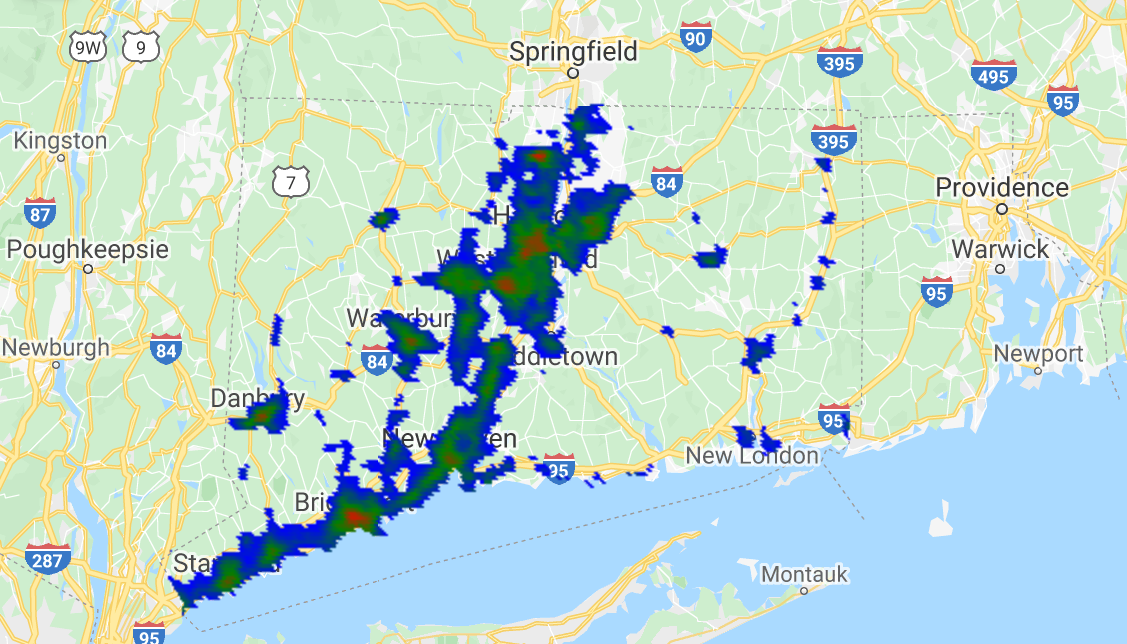## Exporting data

### Exporting a collection to Google Drive, Earth Engine Asset, or Google Cloud

``````Export.image.toDrive({
collection: varImage, description: 'fileName', region: geometry, scale: 1000
});
``````

or `Export.image.toCloudStorage()`, `Export.image.toAsset()`, `Export.table.toDrive()`, `Export.table.toCloudStorage()`, `Export.video.toCloudStorage()`, `Export.video.toDrive()`.

#### Example: Exporting data

1. Define a function to find the mean value of pixels in each feature of a collection.

``````// Function to find mean of pixels in region of interest.
var getRegions = function(image) {
var countyData = ee.FeatureCollection('TIGER/2018/Counties');
// Filter the counties that are in Connecticut.
// This will be the region of interest for the operations.
var roi=countyData.filter(ee.Filter.eq('STATEFP', '09'));
return image.reduceRegions({
// Collection to run operation over.
collection: roi,
// Calculate mean of all pixels in region.
reducer: ee.Reducer.mean(),
// Pixel resolution used for the calculations.
scale: 1000
});
};
``````

2. Load image collection, filter collection to date range, select band of interest, calculate mean of all images in collection, and multiply by scaling factor.

``````var image = ee.ImageCollection('MODIS/MYD13A1')
.filterDate('2002-07-08', '2017-07-08')
.select('NDVI')
.mean()
.multiply(.0001);
// Print final image.
print(image);
// Call function.
var coll = getRegions(image);
``````

``````Export.table.toDrive({
collection: coll,
description: 'NDVI_all',
fileFormat: 'CSV'
});
// Print final collection.
print(coll);
``````

## Bonus: Timelapse example

``````// Timelapse example (based on google API example);
// Create rectangle over Dubai.
var geometry = ee.Geometry.Rectangle([55.1, 25, 55.4, 25.4]);
var allImages = ee.ImageCollection('LANDSAT/LT05/C01/T1_TOA')
// Filter row and path such that they cover Dubai.
.filter(ee.Filter.eq('WRS_PATH', 160))
.filter(ee.Filter.eq('WRS_ROW', 43))
// Filter cloudy scenes.
.filter(ee.Filter.lt('CLOUD_COVER', 30))
// Get required years of imagery.
.filterDate('1984-01-01', '2012-12-30')
// Select 3-band imagery for the video.
.select(['B4', 'B3', 'B2'])
// Make the data 8-bit.
.map(function(image) {
return image.multiply(512).uint8();
});
Export.video.toDrive({
collection: allImages,
// Name of file.
description: 'dubaiTimelapse',
// Quality of video.
dimensions: 720,
// FPS of video.
framesPerSecond: 8,
// Region of export.
region: geometry
});
``````

Dubai timelapse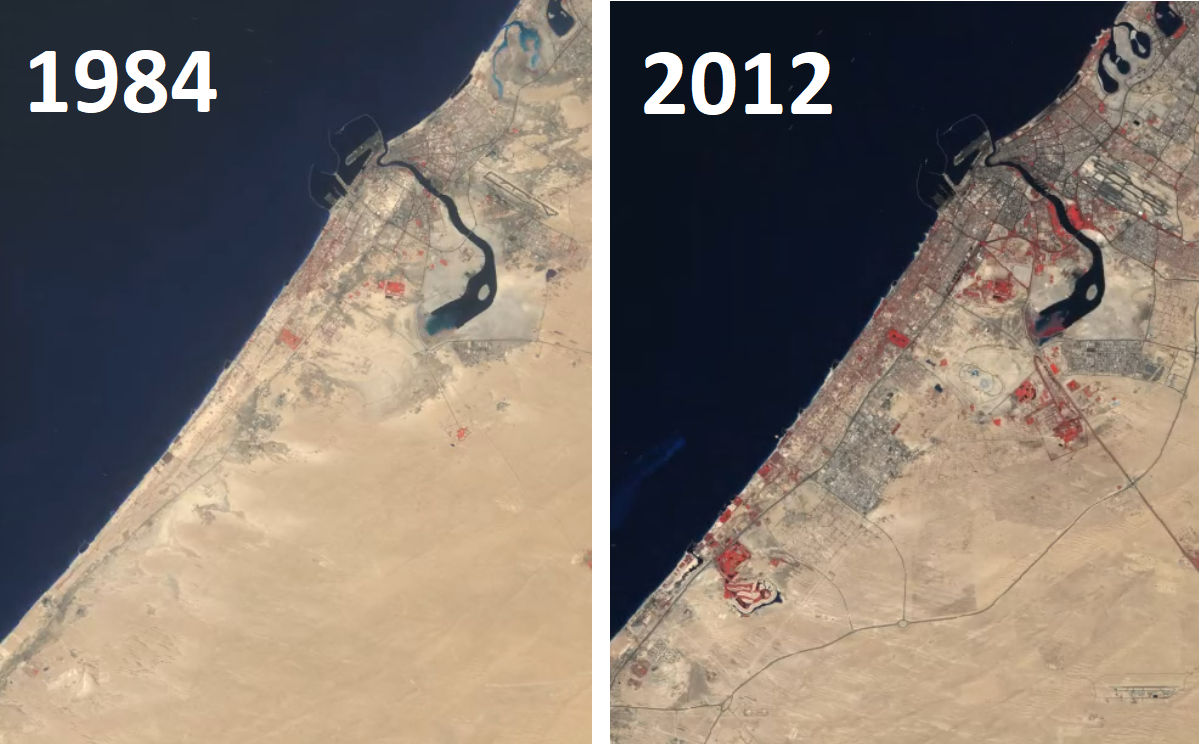## Example applications

What can you do with Google Earth Engine?